CATEGORIES:

# Function. function limit. Fundamental theorems on limits. Infinitely small and infinitely large quantities. The ends

Theoretical questions:

1. Definition limit of function in point. 2. Limit of function in infinite. 3. Infinitely small and infinitely large functions (definition). 4. Basic theorems of limits. 5. Remarkable limits. 6. Definition and properties of infinitely function. 7. Comparing the orders of infinitely small. 8. Application of the infinitesimal to the calculation of limits. 9. One-sided limits. 10. Continuity of function. 11. Classification of points of discontinuity.

Classroom assignments:

1. Using the properties of limits of functions, find the following limits:

1)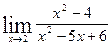; 2); 3)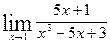;

4)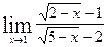; 5);

2. Find limits: 1); 2)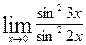.

3. Find limits: 1)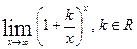; 2)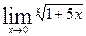;

3);

4. Using equivalents infinite smalls, find the following limits:

1.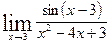2.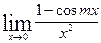3.4.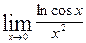5.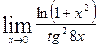6.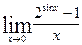5. Investigate continuity of the function and establish character of discontinuity points:

1.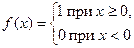2.3.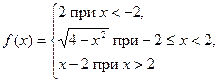.

Homework:

Theoretical material: Derivative of function. Differential of function.

Solve problems:

1. Find limits of functions: а)б)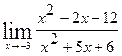в)г)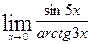д)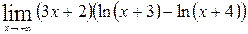2. Investigate continuity of the function, find discontinuity points and establish character of discontinuity points.

а)б)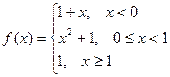.

PRACTICAL CLASS № 6.

Date: 2015-01-02; view: 1362

 <== previous page | next page ==> PRACTICAL CLASS № 3-5. | The derivative of the function. Geometric and mechanical meaning. Table of derivatives. The differential of a function
doclecture.net - lectures - 2014-2023 year. Copyright infringement or personal data (0.013 sec.)